## Delannoy Number

The Delannoy numbers are defined bywhere. They are the number of lattice paths fromtoin which only east (1, 0), north (0, 1), and northeast (1, 1) steps are allowed (i.e,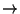,, and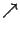).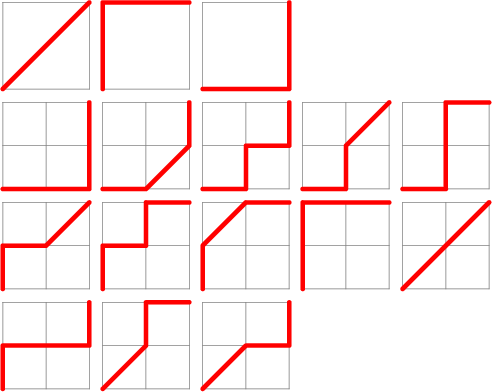For, the Delannoy numbers are the number of king walks''whereis a Legendre Polynomial (Moser 1955, Vardi 1991). Another expression is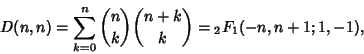where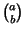is a Binomial Coefficient andis a Hypergeometric Function. The values of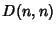for, 2, ... are 3, 13, 63, 321, 1683, 8989, 48639, ... (Sloane's A001850).

The Schröder Numbers bear the same relation to the Delannoy numbers as the Catalan Numbers do to the Binomial Coefficients.

References

Moser, L. King Paths on a Chessboard.'' Math. Gaz. 39, 54, 1955.

Sloane, N. J. A. Sequence A001850/M2942 in An On-Line Version of the Encyclopedia of Integer Sequences.'' http://www.research.att.com/~njas/sequences/eisonline.html and Sloane, N. J. A. and Plouffe, S. The Encyclopedia of Integer Sequences. San Diego: Academic Press, 1995.